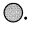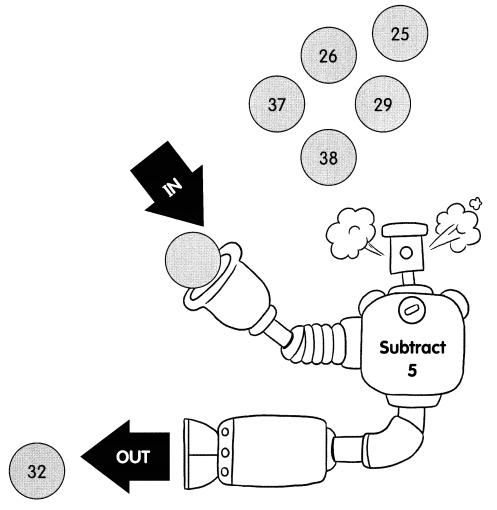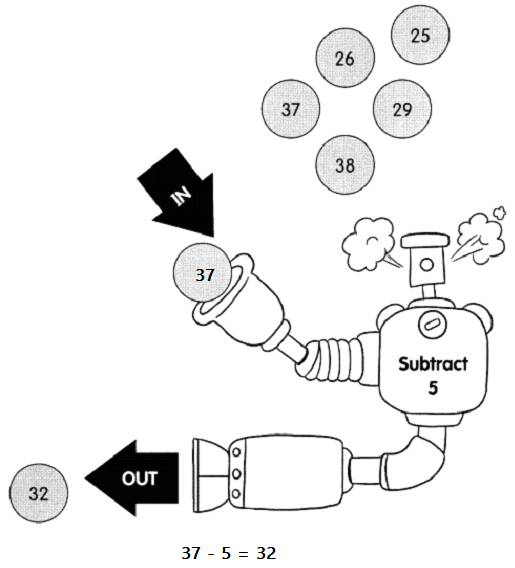# Math in Focus Grade 1 Chapter 13 Practice 3 Answer Key Subtraction Without Regrouping

Practice the problems of Math in Focus Grade 1 Workbook Answer Key Chapter 13 Practice 3 Subtraction Without Regrouping to score better marks in the exam.

## Math in Focus Grade 1 Chapter 13 Practice 3 Answer Key Subtraction Without Regrouping

Subtract by counting back.

Example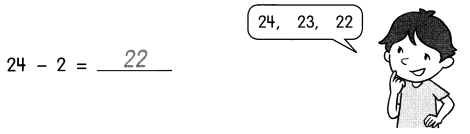Question 1.
27 – 6 = ____Question 2.
35 – 4 = _____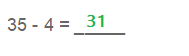Question 3.
38 – 8 = ____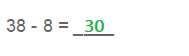Question 4.
24 – 3 = ____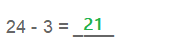Question 5.
39 – 4 = ____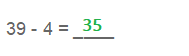Question 6.
39 – 9 = _____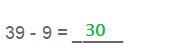Fill in the missing numbers.

Example
37 – 4 = 3 tens 7 ones – 4 ones
= 3 tens 3 ones
= 33

Question 7.
38 – 3 = ______ tens ______ ones – 3 ones
= 3 tens ________ ones
= 3 tens
= _____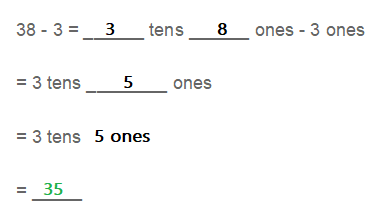Fill in the missing numbers.

Question 8.
26 – 4 = ___ tens 6 ones – ___ ones
= ____ tens 2 ones
= _____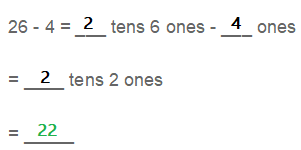Subtract.

Question 9.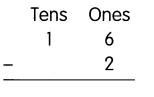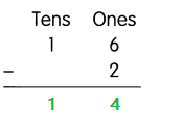Question 10.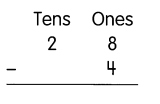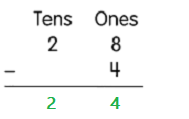Question 11.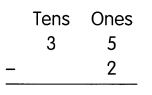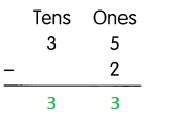Question 12.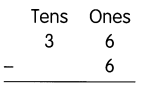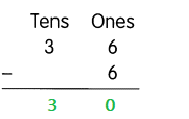Question 13.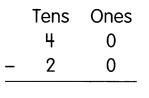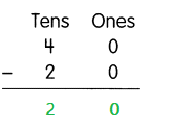Question 14.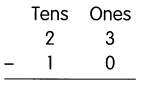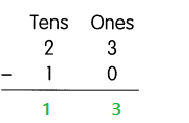Question 15.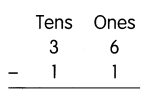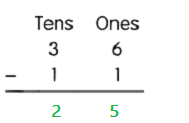Question 16.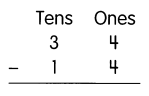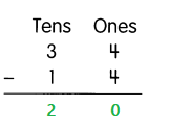Fill in the missing numbers.

Question 17.
29 – 26 = ___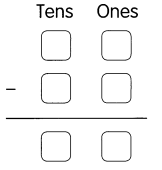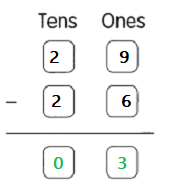Question 18.
38 – 10 = ___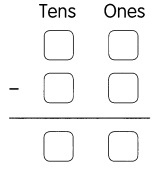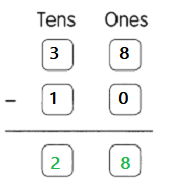Question 19.
31 – 20 = ___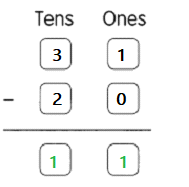Question 20.
27 – 17 = ___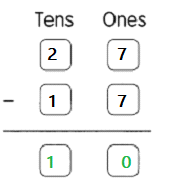Question 21.
36 – 5 = ___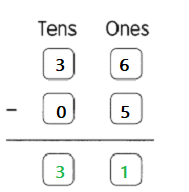Question 22.
38 – 8 = ___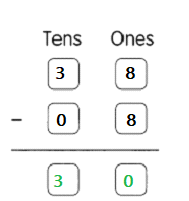Write the correct number in the